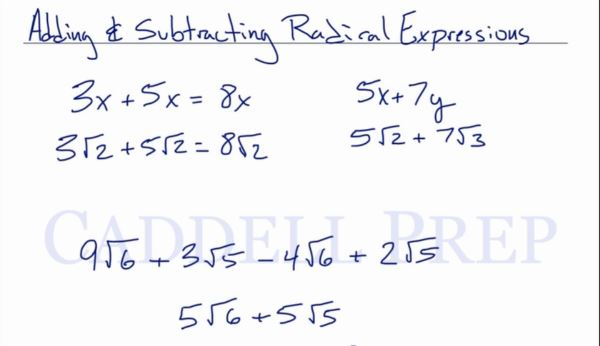In this video, we are going to be adding and subtracting radical expressions. After you finish this lesson, view all of our Algebra 1 lessons and practice problems.

We can add$3x+5x=8x$
and we can do the same to$3\sqrt{2}+5\sqrt{2}=8\sqrt{2}$

Since we can only combine like terms, we know that we cannot add$5x$ and$7y$ together
so we cannot add$5\sqrt{2}$ and$7\sqrt{3}$ together either

For$9\sqrt{6}+3\sqrt{5}-4\sqrt{6}+2\sqrt{5}$,
it would be$5\sqrt{6}+5\sqrt{5}$Let’s look at other examples:$3\sqrt{2}+6\sqrt{18}$$3\sqrt{2}$ would stay like itself, while$6\sqrt{18}$ can be broken into$18\sqrt{2}$
Now that both expressions are like terms, they can be combined into$21\sqrt{2}$$6\sqrt{12}-4\sqrt{45}+2\sqrt{3}-\sqrt{80}$
After simplifying each radical expression, it will be$12\sqrt{3}-12\sqrt{5}+2\sqrt{3}-4\sqrt{5}$
After combining like terms, it will be$14\sqrt{3}-16\sqrt{5}$

Example 1$3\sqrt{18}+3\sqrt{12}+2\sqrt{27}$
First, let’s simplify all these radical expressions$3\sqrt{9}\sqrt{2}+3\sqrt{4}\sqrt{3}+2\sqrt{9}\sqrt{3}$$3\times 3\sqrt{2}+3\times 2\sqrt{3}+2\times 3\sqrt{3}$$9\sqrt{2}+6\sqrt{3}+6\sqrt{3}$
Now, let’s combine the like terms.$9\sqrt{2}+12\sqrt{3}$

Example 2$-3\sqrt{45}+2\sqrt{12}+3\sqrt{6}-3\sqrt{20}$
First, let’s simplify all these radical expressions$-3\sqrt{9}\sqrt{5}+2\sqrt{4}\sqrt{3}+3\sqrt{6}-3\sqrt{4}\sqrt{5}$$-3\times3\sqrt{5}+2\times2\sqrt{3}+3\sqrt{6}-3\times 2\sqrt{5}$$-9\sqrt{5}+4\sqrt{3}+3\sqrt{6}-6\sqrt{5}$
Now, let’s combine the like terms.$-15\sqrt{5}+4\sqrt{3}+3\sqrt{6}$

Video-Lesson Transcript$3x + 5x = 8x$$3 \sqrt{2} + 5 \sqrt{2} = 8 \sqrt{2}$

Only like terms can be added.

Just like if we have$5x + 7y$$5 \sqrt{2} + 7 \sqrt{3}$

it will just stay like this.

They won’t combine since they are different radicals.

So if we have$9 \sqrt{6} + 3 \sqrt{5} - 4 \sqrt{6} + 2 \sqrt{5}$

we can combine like terms, so the answer is$5 \sqrt{6} + 5 \sqrt{5}$

Let’s look at this one$3 \sqrt{2} + 6 \sqrt{18}$

This may look unlike terms so we can’t combine them.

But we can simplify this first.

The first expression can’t be simplified so we simplify the second expression.

Let’s simplify first.$6 \sqrt{18}$$6 \sqrt{9} \sqrt{2}$

I chose these because$9$ is a complete square.$6 \times 3 \sqrt{2}$$18 \sqrt{2}$

Now, let’s our new expressions are$3 \sqrt{2} + 18 \sqrt{2}$

Since they are already like terms, we can combine them now.$21 \sqrt{2}$

So always make sure that they are in their simplest terms before we add them.

Because maybe they can be combined, they are not just in the correct format.

Let’s try this one now.$6 \sqrt{12} - 4 \sqrt{45} + 2 \sqrt{3} - \sqrt{80}$

Let’s simplify all these first.$6 \sqrt{4} \sqrt{3} - 4 \sqrt{9} \sqrt{5} + 2 \sqrt{3} - \sqrt{4} \sqrt{20}$$6 \times 2 \sqrt{3} - 4 \times 3 \sqrt{5} + 2 \sqrt{3} - 2 \sqrt{4} \sqrt{5}$$12 \sqrt{3} - 12 \sqrt{5} + 2 \sqrt{3} - 2 \times 2 \sqrt{5}$$12 \sqrt{3} - 12 \sqrt{5} + 2 \sqrt{3} - 4 \sqrt{5}$

Now, let’s combine the like terms.$14 \sqrt{3} - 16 \sqrt{5}$